Lidar and Radar Fusion in an Urban Air Mobility Scenario

In this example you learn how to use multi-object trackers to track various Unmanned Air Vehicles (UAVs) in an urban environment. You create a scene using the uavScenario object based on building and terrain data available online. You use lidar and radar sensor models to generate synthetic sensor data. Finally, you use various tracking algorithms to estimate the state of all UAVs in the scene.

UAVs are designed for a wide range of operations. Many applications are set in urban environments, such as drone package delivery, air taxis, and power line inspection. The safety of these operations becomes critical as the number of applications grows, making controlling the urban airspace a challenge.

Create an urban air mobility scenario

In this example, you use the terrain and building data of Boulder, CO. The Digital Terrain Elevation Data (DTED) file is downloaded from the "SRTM Void Filled" data set available from the U.S. Geological Survey. The building data, saved in building.mat, is downloaded from OpenStreetMap available online. You create a UAV scenario using this data.

dtedfile = "n39_w106_3arc_v2.dt1";
buildingfile = "building.mat";
scene = createScenario(dtedfile,buildingfile);

Next, you add a few UAVs to the scenario.

To model a package delivery operation, you define a trajectory leaving from the roof of a building and flying to another building. The trajectory is composed of three legs. The quadrotor takes off vertically, then flies toward the next delivery destination, and finally lands vertically on the roof.

waypointsA = [1895 90 20; 1915 108 35; 1900 115 20];
timeA = [0 25 50];
trajA = waypointTrajectory(waypointsA, "TimeOfArrival", timeA, "ReferenceFrame", "ENU", "AutoBank", true);
uavA = uavPlatform("UAV", scene, "Trajectory", trajA, "ReferenceFrame", "ENU");
updateMesh(uavA, "quadrotor", {5}, [0 1 1], eye(4));

You add another UAV to model an air taxi flying by. Its trajectory is linear, slightly descending. You use the fixedwing geometry to model a larger UAV that are suitable to transporting people.

waypointsB = [1940 120 50; 1800 50 20];
timeB = [0 41];
trajB = waypointTrajectory(waypointsB, "TimeOfArrival", timeB, "ReferenceFrame", "ENU", "AutoBank", true);
uavB = uavPlatform("UAV2", scene, "Trajectory", trajB, "ReferenceFrame", "ENU");
updateMesh(uavB, "fixedwing", {10},  [0 1 1], eye(4));

Then you add a quadrotor with a trajectory following the street path. This could represent a UAV inspecting power grid lines for maintenance purposes.

waypointsC = [1950 60 35; 1900 60 35; 1890 80 35];
timeC = linspace(0,41,size(waypointsC,1));
trajC = waypointTrajectory(waypointsC, "TimeOfArrival", timeC, "ReferenceFrame", "ENU", "AutoBank", true);
uavC = uavPlatform("UAV3", scene, "Trajectory", trajC, "ReferenceFrame", "ENU");
updateMesh(uavC, "quadrotor", {5}, [0 1 1], eye(4));

Finally, you add the ego UAV, a UAV responsible for surveilling the scene and tracking different moving platforms.

waypointsD = [1900 140 65; 1910 100 65];
timeD = [0 60];
trajD = waypointTrajectory(waypointsD, "TimeOfArrival", timeD, ...
"ReferenceFrame", "ENU", "AutoBank", true, "AutoPitch", true);
egoUAV = uavPlatform("EgoVehicle", scene, "Trajectory", trajD, "ReferenceFrame", "ENU");
updateMesh(egoUAV, "quadrotor", {5}, [0 0 1], eye(4));

Define UAV sensor suite

Mount sensors on the ego vehicle. You use a lidar puck that is commonly used in automotive . It is a small sensor that can be attached on a quadrotor. You use the following specification for the lidar puck:

• Range resolution: 3 cm

• Maximum range: 100 m

• 360 degrees azimuth span with 0.2° resolution

• 30 degrees elevation span with 2° resolution

• Update rate: 10 Hz

• Mount with a 90° tilt to look down

% Mount a lidar on the quadrotor
lidarOrient = [90 90 0];
lidarSensor = uavLidarPointCloudGenerator("MaxRange",100, ...
"RangeAccuracy", 0.03, ...
"ElevationLimits", [-15 15], ...
"ElevationResolution", 2, ...
"AzimuthLimits", [-180 180], ...
"AzimuthResolution", 0.2, ...
"UpdateRate", 10, ...
"HasOrganizedOutput", false);
lidar = uavSensor("Lidar", egoUAV, lidarSensor, "MountingLocation", [0 0 -3], "MountingAngles",lidarOrient);

• Frequency: 24.45 - 24.65 GHz

• Field of view: 120° azimuth 80° elevation

• Resolution: 2 deg in azimuth, 6° in elevation

• Full scan rate: 1 Hz

• Sensitivity: 0 dBsm at 200 m

Additionally, you configure the radar to output multiple detections per object. Though the radar can output tracks representing point targets, you want to estimate the extent of the target, which is not available with the default track output. Therefore, you set the TargetReportFormat property to Detections so that the radar report crude detections directly.

"FieldOfView",[120 80],...
"UpdateRate", 1,...
'MountingAngles',[0 30 0],...
"HasElevation", true,...
"ElevationResolution", 6,...
"AzimuthResolution", 2, ...
"RangeResolution", 4, ...
"RangeLimits", [0 200],...
'ReferenceRange',200,...
'CenterFrequency',24.55e9,...
'Bandwidth',200e6,...
"TargetReportFormat","Detections",...
"DetectionCoordinates","Sensor rectangular",...
"HasFalseAlarms",false,...
"FalseAlarmRate", 1e-7);

Define tracking system

Lidar point cloud processing

Lidar sensors return point clouds. To fuse the lidar output, the point cloud must be clustered to extract object detections. Segment out the terrain using the segmentGroundSMRF function from Lidar Toolbox. The remaining point cloud is clustered, and a simple threshold is applied to each cluster mean elevation to filter out building detections. Fit each cluster with a cuboid to extract a bounding box detection. The helper class helperLidarDetector available in this example has the implementation details.

Lidar cuboid detections are formatted using the objectDetection object. The measurement state for these detections is $\left[\mathit{x},\text{\hspace{0.17em}}\mathit{y},\text{\hspace{0.17em}}\mathit{z},\text{\hspace{0.17em}}\mathit{L},\text{\hspace{0.17em}}\mathit{W},\text{\hspace{0.17em}}\mathit{H},{\mathit{q}}_{0},\text{\hspace{0.17em}}{\mathit{q}}_{1},\text{\hspace{0.17em}}{\mathit{q}}_{2},\text{\hspace{0.17em}}{\mathit{q}}_{3}\right]$, where:

• $\mathit{x},\text{\hspace{0.17em}}\mathit{y},\text{\hspace{0.17em}}\mathit{z}$ are the cuboid center coordinates along the East, North, and Up axes of the scenario, respectively.

• $\mathit{L},\text{\hspace{0.17em}}\mathit{W},\text{\hspace{0.17em}}\mathit{H}$ are the length, width, and height of the cuboid, respectively.

• $\text{\hspace{0.17em}}{\mathit{q}\text{\hspace{0.17em}}=\text{\hspace{0.17em}}\mathit{q}}_{0}+{\text{\hspace{0.17em}}\mathit{q}}_{1}.\mathit{i}+\text{\hspace{0.17em}}{\mathit{q}}_{2}.\mathit{j}+\text{\hspace{0.17em}}\text{\hspace{0.17em}}{\mathit{q}}_{3}.\mathit{k}$ is the quaternion defining the orientation of the cuboid with respect to the ENU axes.

lidarDetector = helperLidarDetector(scene)
lidarDetector =
helperLidarDetector with properties:

GridResolution: 1.5000
SegmentationMinDistance: 5
MinDetectionsPerCluster: 2
MinZDistanceCluster: 20

Lidar tracker

You use a point target tracker, trackerJPDA, to track the lidar bounding box detections. A point tracker assumes that each UAV can generate at most one detection per sensor scan. This assumption is valid because you have clustered the point cloud into cuboids. To setup a tracker, you need to define the motion model and the measurement model. In this example, you model the dynamics of UAVs using an augmented constant velocity model. The constant velocity model is sufficient to track trajectories consisting of straight flight legs or slowly varying segments. Moreover, assume the orientation of the UAV is constant and assume the dimensions of the UAVs are constant. As a result, the track state and state transition equations are $\mathit{X}=\text{\hspace{0.17em}}\left[\mathit{x},{\mathit{v}}_{\mathit{x}}\text{\hspace{0.17em}},\text{\hspace{0.17em}}\mathit{y},\text{\hspace{0.17em}}{\mathit{v}}_{\mathit{y}}\text{\hspace{0.17em}},\text{\hspace{0.17em}}\mathit{z},\text{\hspace{0.17em}}{\mathit{v}}_{\mathit{z}\text{\hspace{0.17em}}}\text{\hspace{0.17em}},\mathit{L},\text{\hspace{0.17em}}\mathit{W},\text{\hspace{0.17em}}\mathit{H},{\mathit{q}}_{0},{\mathit{q}}_{1},{\mathit{q}}_{2},{\mathit{q}}_{3}\right]$ and

${\mathit{X}}_{\mathit{k}+1}=\left[\begin{array}{ccc}\left[\begin{array}{cccccc}1& {\mathit{t}}_{\mathit{s}}& 0& .& .& 0\\ 0& 1& 0& .& & .\\ .& .& 1& {\mathit{t}}_{\mathit{s}}& .& .\\ .& & .& 1& 0& 0\\ .& & & .& 1& {\mathit{t}}_{\mathit{s}}\\ 0& .& .& .& 0& 1\end{array}\right]& {0}_{3}& {0}_{3}\\ {0}_{3}& {\mathit{I}}_{3}& {0}_{3\mathrm{x4}}\\ {0}_{3}& {0}_{4\mathrm{x3}}& {\mathit{I}}_{4}\end{array}\right]{\mathit{X}}_{\mathit{k}}\text{\hspace{0.17em}}+{\mathit{Q}}_{\mathit{k}}$

Here, ${\mathit{v}}_{\mathit{x}}$, ${\mathit{v}}_{\mathit{y}}$, ${\mathit{v}}_{\mathit{z}\text{\hspace{0.17em}}}$ are the cuboid velocity vector coordinates along the scenario ENU axes. You track orientation using a quaternion because of the discontinuity of Euler angles when using tracking filters. ${\mathit{t}}_{\mathit{s}}$ , the time interval between updates k and k+1, is equal to 0.1 seconds. Lastly, ${\mathit{Q}}_{\mathit{k}}$ is the additive process noise that captures the modeling inaccuracy.

The inner transition matrix corresponds to the constant velocity model. You define an augmented state version of constvel and cvmeas to account for the additional constant states. The details are implemented in the supporting functions initLidarFilter, augmentedConstvel, augmentedConstvelJac, augmentedCVmeas, and augmentedCVMeasJac in the end of the example.

lidarJPDA = trackerJPDA('TrackerIndex',2,...
'AssignmentThreshold',[70 150],...
'ClutterDensity',1e-16,...
'DetectionProbability',0.99,...
'DeletionThreshold',[10 10],... Delete lidar track if missed for 1 second
'ConfirmationThreshold',[4 5],...
'FilterInitializationFcn',@initLidarFilter)
lidarJPDA =
trackerJPDA with properties:

TrackerIndex: 2
FilterInitializationFcn: @initLidarFilter
MaxNumEvents: Inf
EventGenerationFcn: 'jpdaEvents'
MaxNumTracks: 100
MaxNumDetections: Inf
MaxNumSensors: 20
TimeTolerance: 1.0000e-05

OOSMHandling: 'Terminate'

AssignmentThreshold: [70 150]
InitializationThreshold: 0
DetectionProbability: 0.9900
ClutterDensity: 1.0000e-16

TrackLogic: 'History'
ConfirmationThreshold: [4 5]
DeletionThreshold: [10 10]
HitMissThreshold: 0.2000

HasCostMatrixInput: false
HasDetectableTrackIDsInput: false
StateParameters: [1×1 struct]

NumTracks: 0
NumConfirmedTracks: 0

In this example, you assume the radar returns are preprocessed such that only returns from moving objects are preserved, that is no returns from the ground or the buildings. The radar measurement state is $\left[\mathit{x},{\mathit{v}}_{\mathit{x}\text{\hspace{0.17em}}},\mathit{y},{\mathit{v}}_{\mathit{y}\text{\hspace{0.17em}}},\mathit{z},{\mathit{v}}_{\mathit{z}}\right]$. The radar resolution is fine enough to generate multiple returns per UAV target and its detections should not be fed directly to a point target tracker. There are two possible approaches to track with the high-resolution radar detections. One way, you can cluster the detections and augment the state with dimensions and orientation constants as done previously with the lidar cuboids. On the other way, you can feed the detections to an extended target tracker adopted in this example by using a GGIW-PHD tracker. This tracker estimates the extent of each target using an inverse Wishart distribution, whose expectation is a 3-by-3 positive definite matrix, representing the extent of a target as a 3D ellipse. This second approach is preferable because there aren't too many detections per object and clustering is less accurate than extended-target tracking

To create a GGIW-PHD tracker, you first define the tracking sensor configuration for each sensor reporting to the tracker. In this case, you only need to define the configuration for one radar. When the radar mounting platform is moving, you need to update this configuration with the current radar pose before each tracker step. Next, you define a filter initialization function based on the sensor configuration. Finally, you construct a trackerPHD object and increase the partitioning threshold to capture the dimensions of objects tracked in this example. The implementation details are shown at the end of the example in the supporting function createRadarTracker.

trackerPHD with properties:

TrackerIndex: 1
SensorConfigurations: {[1×1 trackingSensorConfiguration]}
PartitioningFcn: @(dets)partitionDetections(dets,threshold(1),threshold(2),'Distance','euclidean')
MaxNumSensors: 20
MaxNumTracks: 1000

AssignmentThreshold: 50
BirthRate: 1.0000e-03
DeathRate: 1.0000e-06

ExtractionThreshold: 0.8000
ConfirmationThreshold: 0.9900
DeletionThreshold: 0.1000
MergingThreshold: 50
LabelingThresholds: [1.0100 0.0100 0]

StateParameters: [1×1 struct]
HasSensorConfigurationsInput: true
NumTracks: 0
NumConfirmedTracks: 0

Track fusion

The final step in creating the tracking system is to define a track fuser object to fuse lidar tracks and radar tracks. You use the 13-dimensional state of lidar tracks as the fused state definition.

'IsInitializingCentralTracks',true);

lidarConfig = fuserSourceConfiguration('SourceIndex',2,...
'IsInitializingCentralTracks',true);

'ProcessNoise',blkdiag(2*eye(6),1*eye(3),0.2*eye(4)),...
'AssignmentThreshold',200,...
'ConfirmationThreshold',[4 5],...
'DeletionThreshold',[5 5],...
'StateFusion','Cross',...
'StateTransitionFcn',@augmentedConstvel,...
'StateTransitionJacobianFcn',@augmentedConstvelJac);

Visualization

You use a helper class to visualize the scenario. This helper class utilizes the uavScenario visualization capabilities and the theaterPlot plotter to represent detection and track information.

The display is divided into 5 tiles, showing respectively, the overall 3D scene, three chase cameras for three UAVs, and the legend.

viewer = helperUAVDisplay(scene);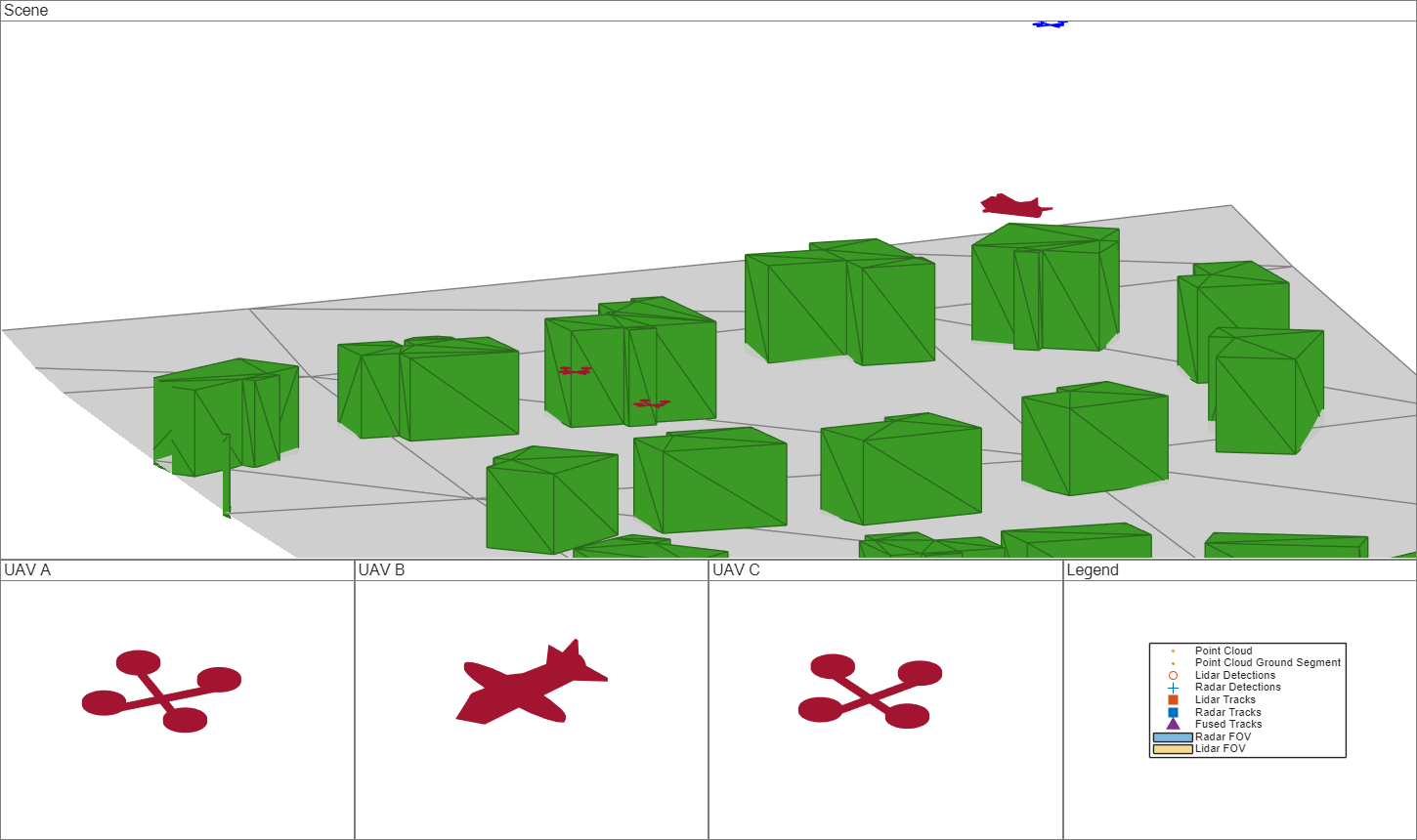Simulate the scenario

You run the scenario and visualize the results of the tracking system. The true pose of each target as well as the radar, lidar, and fused tracks are saved for off-line metric analysis.

setup(scene);
s = rng;
rng(2021);

numSteps = scene.StopTime*scene.UpdateRate;
truthlog = cell(1,numSteps);
lidarlog = cell(1,numSteps);
fusedlog = cell(1,numSteps);
logCount = 0;

time = scene.CurrentTime;

% Track with lidar
[lidardets, lidarTracks, nonGroundCloud, groundCloud] = updateLidarTracker(lidar,lidarDetector, lidarJPDA, egoPose);

% Fuse lidar and radar tracks
if isLocked(fuser) || ~isempty(radarTracks) || ~isempty(lidarTracks)
else
fusedTracks = objectTrack.empty;
end

% Save log
logCount = logCount + 1;
lidarlog{logCount} = lidarTracks;
fusedlog{logCount} = fusedTracks;
truthlog{logCount} = logTargetTruth(scene.Platforms(1:3));

% Update figure
end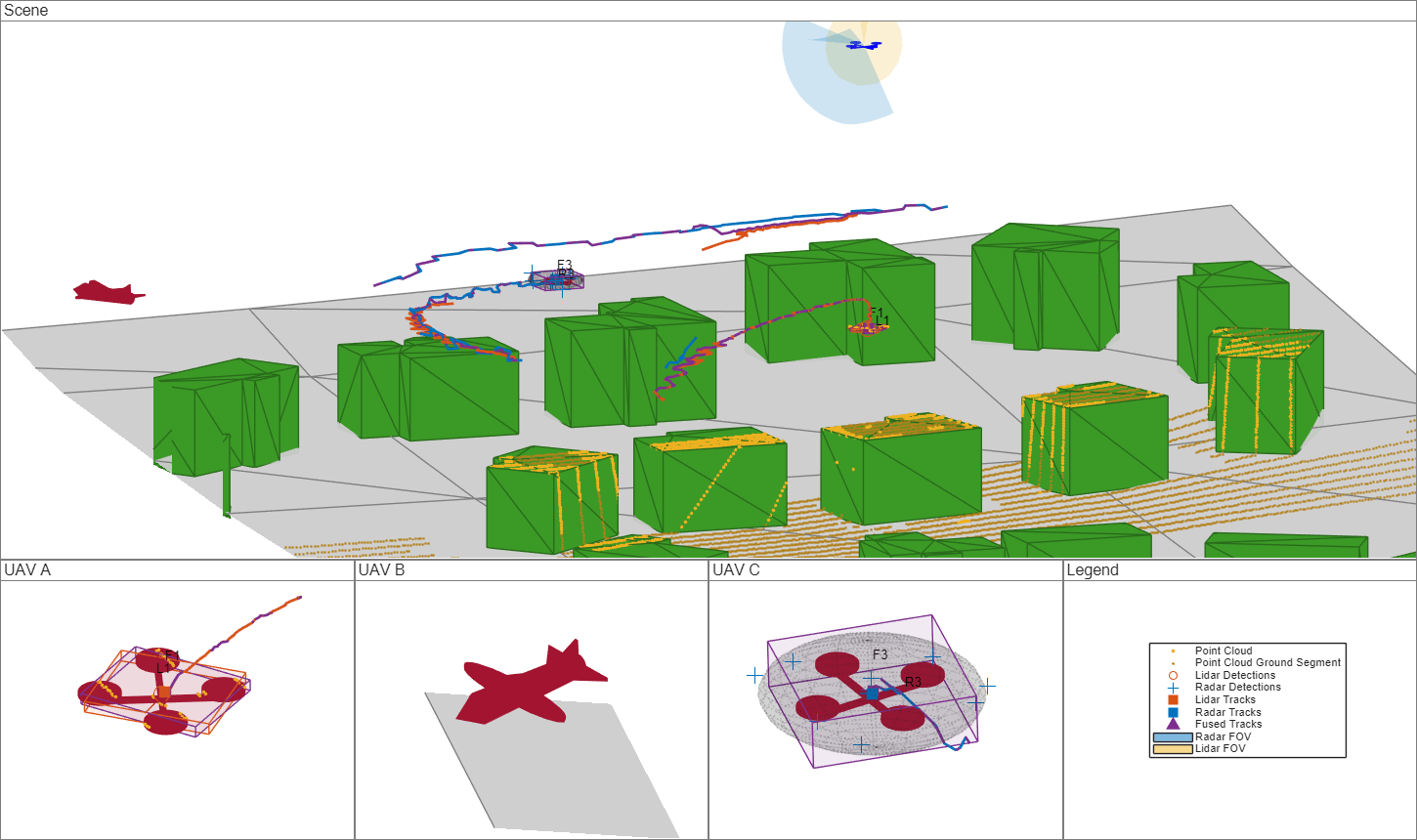Based on the visualization results, you perform an initial qualitative assessment of the tracking performance. The display at the end of the scenario shows that all three UAVs were well tracked by the ego. With the current sensor suite configuration, lidar tracks were only established partially due to the limited coverage of the lidar sensor. The wider field of view of the radar allowed establishing radar tracks more consistently in this scenario.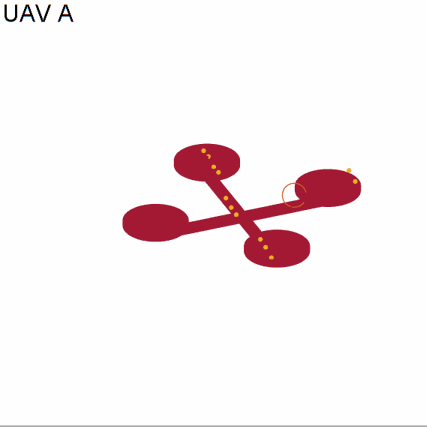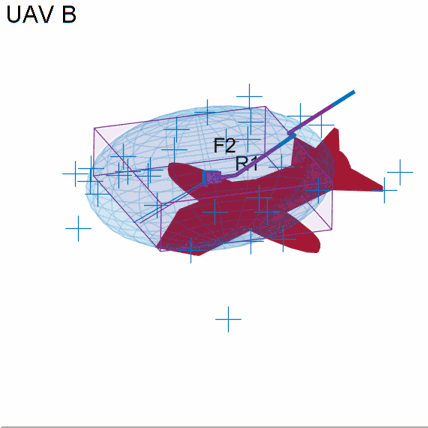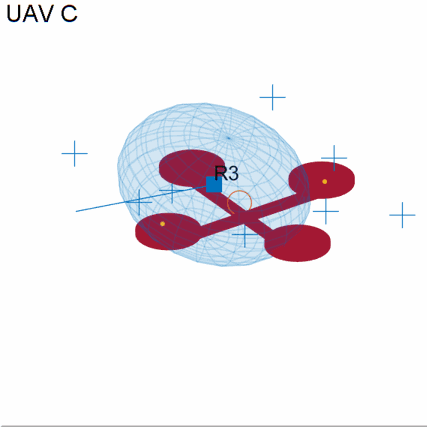The three animated GIFs above show parts of the chase views. You can see that the quality of lidar tracks (orange box) is affected by the geometry of the scenario. UAV A (left) is illuminated by the lidar (shown in yellow) almost directly from above. This enables the tracker to capture the full extent of the drone over time. However, UAV C (right) is partially seen by the radar which leads to underestimating the size of the drone. Also, the estimated centroid periodically oscillates around the true drone center. The larger fixed-wing UAV (middle) generates many lidar points. Thus, the tracker can detect and track the full extent of the target once it has completely entered the field of view of the lidar. In all three cases, the radar, shown in blue, provides more accurate information of the target extent. As a result, the fused track box (in purple) is more closely capturing the extent of each UAV. However, the radar returns are less accurate in position. Radar tracks show more position bias and poorer orientation estimate.

Tracking metrics

In this section you analyze the performance of the tracking system using the GOSPA tracking metric. You first define the distance function which quantifies the error between track and truth using a scalar value. A lower GOSPA value means an overall better performance.

gospaR = trackGOSPAMetric('Distance','custom','DistanceFcn',@metricDistance);
gospaL = clone(gospaR);
gospaF = clone(gospaR);

gospaLidar = zeros(1,numSteps);
gospaFused = zeros(1,numSteps);

for i=1:numSteps
truth = truthlog{i};
gospaLidar(i) = gospaL(lidarlog{i},truth);
gospaFused(i) = gospaF(fusedlog{i},truth);
end

figure
hold on
grid on
plot(gospaLidar,'Color',viewer.LidarColor,'LineWidth',2);
plot(gospaFused,'Color',viewer.FusedColor,'LineWidth',2);
xlabel('Steps')
ylabel('GOSPA')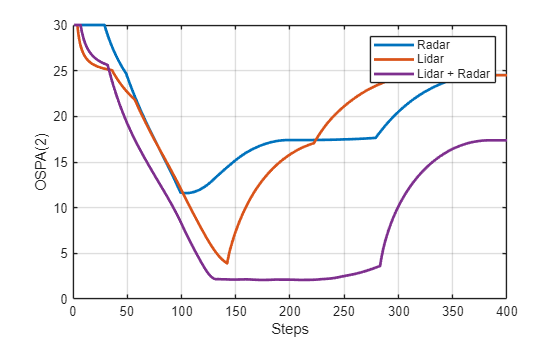You analyze the overall system performance. Each tracker is penalized for not tracking any of the UAVs even if the target UAV is outside of the sensor coverage. This shows improved performance when fusing lidar and radar due to the added surveillance area. This is particularly noticeable at the end of the simulation where two targets are tracked, one by radar and the other by lidar, but both are tracked by the fuser. Additionally, you can see that the fused GOSPA is below the minimum of lidar and radar GOSPA, showing the fused track has better quality than each individual track.

% clean up
removeCustomTerrain("southboulder");
rng(s);

Summary

In this example, you have learned how to model a UAV-borne lidar and radar tracking system and tested it on an urban air mobility scenario. You used the uavScenario object to create a realistic urban environment with terrain and buildings. You generated synthetic sensor data to test a complete tracking system chain, involving point cloud processing, point target and extended target tracking, and track fusion.

Supporting Functions

createScenario creates the uavScenario using the OpenStreetMap terrain and building mesh data

function scene = createScenario(dtedfile,buildingfile)

try
catch
end

minHeight = 1.6925e+03;
latlonCenter = [39.9786 -105.2882 minHeight];
scene = uavScenario("UpdateRate",10,"StopTime",40,...
"ReferenceLocation",latlonCenter);

sceneXLim = [1800 2000];
sceneYLim = [0 200];
scene.addMesh("terrain", {"southboulder", sceneXLim, sceneYLim},[0 0 0]);

for idx = 1:numel(buildings)-1
v = buildings{idx}.Vertices;
v(:,3) = v(:,3) - minHeight;
rangeVMin = min(v);
rangeVMax = max(v);
if rangeVMin(1) > sceneXLim(1) && rangeVMax(1) < sceneXLim(2) &&...
rangeVMin(2) > sceneYLim(1) && rangeVMax(2) < sceneYLim(2)
end
end

end

% Create sensor configuration for trackerPHD
sensorLimits = [-fov(1)/2 fov(1)/2; -fov(2)/2 fov(2)/2; 0 inf];

sensorOrient = rotmat(quaternion(radar.MountingAngles, 'eulerd', 'ZYX', 'frame'),'frame');

% Specify frame info of radar with respect to UAV
sensorTransformParameters(1) = struct('Frame','Spherical',...
'OriginPosition', sensorPos,...
'OriginVelocity', zeros(3,1),...% Sensor does not move relative to ego
'Orientation', sensorOrient,...
'IsParentToChild',true,...% Frame rotation is supplied as orientation
'HasElevation',true,...
'HasVelocity',false);

% Specify frame info of UAV with respect to scene
sensorTransformParameters(2) = struct('Frame','Rectangular',...
'OriginPosition', egoPose(1:3),...
'OriginVelocity', egoPose(4:6),...
'Orientation', rotmat(quaternion(egoPose(10:13)),'Frame'),...
'IsParentToChild',true,...
'HasElevation',true,...
'HasVelocity',false);

'IsValidTime', true,...
'SensorLimits',sensorLimits,...
'SensorResolution', sensorResolution,...
'DetectionProbability',Pd,...
'ClutterDensity', Kc,...
'SensorTransformFcn',@cvmeas,...
'SensorTransformParameters', sensorTransformParameters);

% Threshold for partitioning
threshold = [3 16];
tracker = trackerPHD('TrackerIndex',1,...
'HasSensorConfigurationsInput',true,...
'BirthRate',1e-3,...
'AssignmentThreshold',50,...% Minimum negative log-likelihood of a detection cell to add birth components
'ExtractionThreshold',0.80,...% Weight threshold of a filter component to be declared a track
'ConfirmationThreshold',0.99,...% Weight threshold of a filter component to be declared a confirmed track
'MergingThreshold',50,...% Threshold to merge components
'DeletionThreshold',0.1,...% Threshold to delete components
'LabelingThresholds',[1.01 0.01 0],...% This translates to no track-splitting. Read LabelingThresholds help
'PartitioningFcn',@(dets) partitionDetections(dets, threshold(1),threshold(2),'Distance','euclidean'));
end

initRadarfilter implements the GGIW-PHD filter used by the trackerPHD object. This filter is used during a tracker update to 1) initialize new birth components in the density and 2) initialize new component from detection partitions.

if nargin == 0

% Process noise
sigP = 0.2;
sigV = 1;
Q = diag([sigP, sigV, sigP, sigV, sigP, sigV].^2);

phd = ggiwphd(zeros(6,0),repmat(eye(6),[1 1 0]),...
'ScaleMatrices',zeros(3,3,0),...
'MaxNumComponents',1000,...
'ProcessNoise',Q,...
'MeasurementFcn', @cvmeas,...
'MeasurementJacobianFcn', @cvmeasjac,...
'PositionIndex', [1 3 5],...
'ExtentRotationFcn', @(x,dT)eye(3,class(x)),...
'StateTransitionFcn', @constvel,...
'StateTransitionJacobianFcn', @constveljac);

else %nargin == 1
% ------------------
% 1) Configure Gaussian mixture
% 2) Configure Inverse Wishart mixture
% 3) Configure Gamma mixture
% -----------------

%% 1) Configure Gaussian mixture
meanDetection = detectionPartition{1};
n = numel(detectionPartition);

% Collect all measurements and measurement noises.
allDets = [detectionPartition{:}];
zAll = horzcat(allDets.Measurement);
RAll = cat(3,allDets.MeasurementNoise);

% Specify mean noise and measurement
z = mean(zAll,2);
R = mean(RAll,3);
meanDetection.Measurement = z;
meanDetection.MeasurementNoise = R;

% Parse mean detection for position and velocity covariance.

% Create a constant velocity state and covariance
states = zeros(6,1);
covariances = zeros(6,6);
states(1:2:end) = posMeas;
states(2:2:end) = velMeas;
covariances(1:2:end,1:2:end) = posCov;
covariances(2:2:end,2:2:end) = 10*eye(3);

% process noise
sigP = 0.2;
sigV = 1;
Q = diag([sigP, sigV, sigP, sigV, sigP, sigV].^2);

%% 2) Configure Inverse Wishart mixture parameters
% The extent is set to the spread of the measurements in positional-space.
e = zAll - z;
Z = e*e'/n + R;
dof = 150;
% Measurement Jacobian
p = detectionPartition{1}.MeasurementParameters;
H = cvmeasjac(states,p);

Bk = H(:,1:2:end);
Bk2 = eye(3)/Bk;
V = (dof-4)*Bk2*Z*Bk2';

% Configure Gamma mixture parameters such that the standard deviation
% of the number of detections is n/4
alpha = 16; % shape
beta = 16/n; % rate

phd = ggiwphd(...
... Gaussian parameters
states,covariances,...
'ProcessNoise',Q,...
'MeasurementFcn', @cvmeas,...
'MeasurementJacobianFcn' , @cvmeasjac,...
'StateTransitionFcn', @constvel,...
'StateTransitionJacobianFcn' , @constveljac,...
'PositionIndex'  ,[1 3 5],...
'ExtentRotationFcn' , @(x,dT) eye(3),...
... Inverse Wishart parameters
'DegreesOfFreedom',dof,...
'ScaleMatrices',V,...
'TemporalDecay',150,...
... Gamma parameters
'Shapes',alpha,'Rates',beta,...
'GammaForgettingFactors',1.05);
end

end

formatPHDTracks formats the elliptical GGIW-PHD tracks into rectangular augmented state tracks for track fusion. convertExtendedTrack returns state and state covariance of augmented rectangular state. The Inverse Wishart random matrix eigen values are used to derive rectangular box dimensions. The eigen vectors provide with the orientation quaternion. In this example, you use an arbitrary covariance for radar track dimension and orientation, which is often sufficient for tracking.

function tracksout = formatPHDTracks(tracksin)
% Convert track struct from ggiwphd to objectTrack with state definition
% [x y z vx vy vz L W H q0 q1 q2 q3]
N = numel(tracksin);
tracksout = repmat(objectTrack,N,1);
for i=1:N
tracksout(i) = objectTrack(tracksin(i));
[state, statecov] = convertExtendedTrack(tracksin(i));
tracksout(i).State = state;
tracksout(i).StateCovariance = statecov;
end
end

function [state, statecov] = convertExtendedTrack(track)
% Augment the state with the extent information

extent = track.Extent;
[V,D] = eig(extent);
% Choose L > W > H. Use 1.5 sigma as the dimension
[dims, idx] = sort(1.5*sqrt(diag(D)),'descend');
V = V(:,idx);
q = quaternion(V,'rotmat','frame');
q = q./norm(q);
[q1, q2, q3, q4] = parts(q);
state = [track.State; dims(:); q1 ; q2 ; q3 ; q4 ];
statecov = blkdiag(track.StateCovariance, 4*eye(3), 4*eye(4));

end

updateRadarTracker updates the radar tracking chain. The function first reads the current radar returns. Then the radar returns are passed to the GGIW-PHD tracker after updating its sensor configuration with the current pose of the ego drone.

if mod(time,1) ~= 0
end
% Update radar sensor configuration for the tracker
configs{1}.SensorTransformParameters(2).OriginPosition = egoPose(1:3);
configs{1}.SensorTransformParameters(2).OriginVelocity = egoPose(4:6);
configs{1}.SensorTransformParameters(2).Orientation = rotmat(quaternion(egoPose(10:13)),'frame');
else
end
end

updateLidarTracker updates the lidar tracking chain. The function first reads the current point cloud output from the lidar sensor. Then the point cloud is processed to extract object detections. Finally, these detections are passed to the point target tracker.

function [lidardets, lidarTracks,nonGroundCloud, groundCloud] = updateLidarTracker(lidar, lidarDetector,lidarJPDA, egoPose)
% lidar is always updated
[lidardets,nonGroundCloud, groundCloud] = lidarDetector(egoPose, ptCloud,time);
if isLocked(lidarJPDA) || ~isempty(lidardets)
lidarTracks = lidarJPDA(lidardets,time);
else
lidarTracks = objectTrack.empty;
end
end

initLidarFilter initializes filter for the lidar tracker. The initial track state is derived from the detection position measurement. Velocity is set to 0 with a large covariance to allow future detections to be associated to the track. Augmented state motion model, measurement functions, and jacobians are also defined below.

function ekf = initLidarFilter(detection)

% Lidar measurement: [x y z L W H q0 q1 q2 q3]
meas = detection.Measurement;
initState = [meas(1);0;meas(2);0;meas(3);0; meas(4:6);meas(7:10) ];
initStateCovariance = blkdiag(100*eye(6), 100*eye(3), eye(4));

% Process noise standard deviations
sigP = 1;
sigV = 2;
sigD = 0.5; % Dimensions are constant but partially observed
sigQ = 0.5;

Q = diag([sigP, sigV, sigP, sigV, sigP, sigV, sigD, sigD, sigD, sigQ, sigQ, sigQ, sigQ].^2);

ekf = trackingEKF('State',initState,...
'StateCovariance',initStateCovariance,...
'ProcessNoise',Q,...
'StateTransitionFcn',@augmentedConstvel,...
'StateTransitionJacobianFcn',@augmentedConstvelJac,...
'MeasurementFcn',@augmentedCVmeas,...
'MeasurementJacobianFcn',@augmentedCVmeasJac);
end

function stateOut = augmentedConstvel(state, dt)
% Augmented state for constant velocity
stateOut = constvel(state(1:6,:),dt);
stateOut = vertcat(stateOut,state(7:end,:));
% Normalize quaternion in the prediction stage
idx = 10:13;
qparts = stateOut(idx);
n = sqrt(sum(qparts.^2));
qparts = qparts./n;
if qparts(1) < 0
stateOut(idx) = -qparts;
else
stateOut(idx) = qparts;
end
end

function jacobian = augmentedConstvelJac(state,varargin)
jacobian = constveljac(state(1:6,:),varargin{:});
jacobian = blkdiag(jacobian, eye(7));
end

function measurements = augmentedCVmeas(state)
measurements = cvmeas(state(1:6,:));
measurements = [measurements; state(7:9,:); state(10:13,:)];
end

function jacobian = augmentedCVmeasJac(state,varargin)
jacobian = cvmeasjac(state(1:6,:),varargin{:});
jacobian = blkdiag(jacobian, eye(7));
end

logTargetTruth logs true pose and dimensions throughout the simulation for performance analysis.

function logEntry = logTargetTruth(targets)
n = numel(targets);
targetPoses = repmat(struct('Position',[],'Velocity',[],'Dimension',[],'Orientation',[]),1,n);
uavDimensions = [5 5 0.3 ; 9.8 8.8 2.8; 5 5 0.3];
for i=1:n
targetPoses(i).Position = pose(1:3);
targetPoses(i).Velocity = pose(4:6);
targetPoses(i).Dimension = uavDimensions(i,:);
targetPoses(i).Orientation = pose(10:13);
end
logEntry = targetPoses;
end

metricDistance defines a custom distance for GOSPA. This distance incorporates errors in position, velocity, dimension, and orientation of the tracks.

function out = metricDistance(track,truth)
positionIdx = [1 3 5];
velIdx = [2 4 6];
dimIdx = 7:9;
qIdx = 10:13;

trackpos = track.State(positionIdx);
trackvel = track.State(velIdx);
trackdim = track.State(dimIdx);
trackq = quaternion(track.State(qIdx)');

truepos = truth.Position;
truevel = truth.Velocity;
truedim = truth.Dimension;
trueq = quaternion(truth.Orientation);

errpos = truepos(:) - trackpos(:);
errvel = truevel(:) - trackvel(:);
errdim = truedim(:) - trackdim(:);

% Weights expressed as inverse of the desired accuracy
posw = 1/0.2; %m^-1
velw = 1/2; % (m/s) ^-1
dimw = 1/4; % m^-1
orw = 1/20; % deg^-1

distPos = sqrt(errpos'*errpos);
distVel = sqrt(errvel'*errvel);
distdim = sqrt(errdim'*errdim);